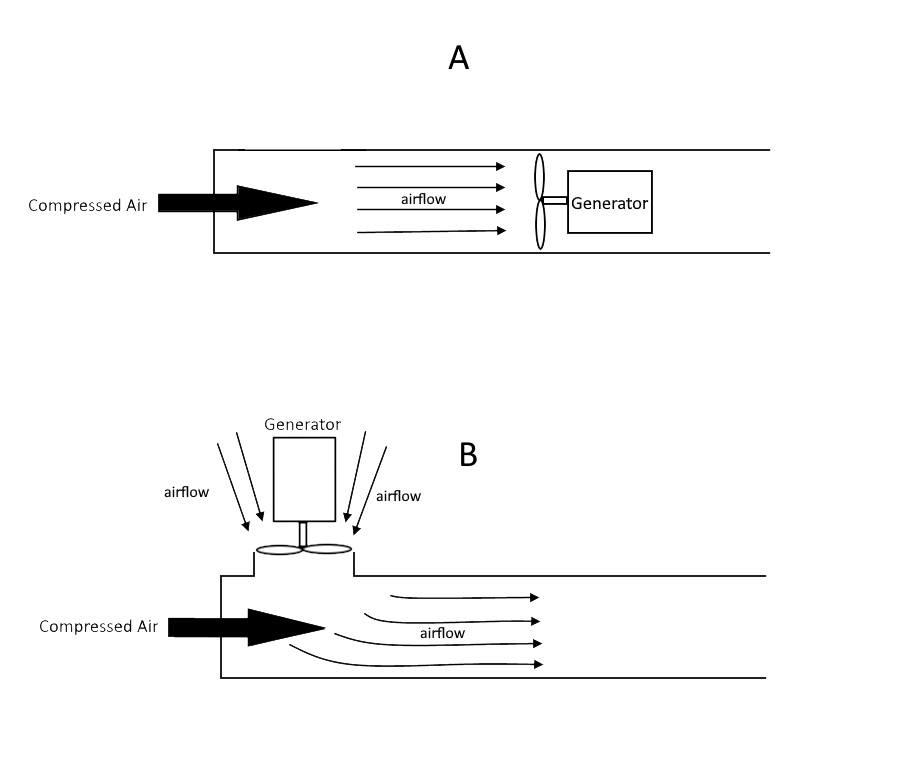# Venturi Ejector Air Flow Mechanics

• mellotango

#### mellotango

Hi,

I am trying to figure out the strength of the various type of airflow for my Venturi ejector device. Please refer to my diagram that illustrates scenario A or scenario B. The SAME amount of compressed air is injected for both scenario A and B. For simplicity, I have added to an electrical generator to measure the strength or power of the airflow.

1. I would like to know which scenario (A or B) would generate more power with the generator.

2. And by what factor would the difference in power level of the airflows between A and B?

I am trying determine if the power of sucked air flow is better, equal or worse than the power of direct air flow. If any engineers can help me out with the calculations, I would greatly appreciate it.

p.s. sorry, i made a quick change to drawing ALast edited:

Ejectors come in various sizes and performance levels. A good place to start is Fox Valve: https://www.foxvalve.com/. Then dive into Chemical Engineer's Handbook by Perry and Chilton. They have a good section on ejectors, complete with performance curves. I see the current edition has a slightly different title: Perry's Chemical Engineer's Handbook.

Using the information from the above sources, you can design an ejector for a particular set of pressures and flow rates, then calculate the amount of energy available for a turbine.

Some hints:
Momentum is conserved.
Kinetic energy is not conserved.
Nobody does this in the real world, there must be a reason why. Can you figure it out?
You can learn a lot by working one or two examples.

•russ_watters
Some hints:
Momentum is conserved.
Kinetic energy is not conserved.
Nobody does this in the real world, there must be a reason why. Can you figure it out?
You can learn a lot by working one or two examples.

Thanks for your answer. I am trying to see, if injecting the same amounting of compressed air energy to A and B, I would be able to obtain the same airflow power output in scenario B. For example, if the turbine generator displayed 100 Kwh output in A, what would the power output display in B be?

Since the compressed air energy provided to both A and B is the same, will the airflow power generated by A nd B be the same, and if not (as witnessed by the turbine generator), where has the loss of energy occured?

Thanks for your answer. I am trying to see, if injecting the same amounting of compressed air energy to A and B, I would be able to obtain the same airflow power output in scenario B. For example, if the turbine generator displayed 100 Kwh output in A, what would the power output display in B be?

Since the compressed air energy provided to both A and B is the same, will the airflow power generated by A nd B be the same, and if not (as witnessed by the turbine generator), where has the loss of energy occured?
It would become clearer if the diagrams were drawn properly and the process described properly. You describe the input air as "compressed", yet you have no nozzles or diffusers, just straight tubes. It's much easier to visualize the energy changes if you can see where the air is speeding up or slowing down, and where the pressure is dropping. Hint: if the injected air is "compressed" in scenario B, it flows out the top hole, not in.

It would become clearer if the diagrams were drawn properly and the process described properly. You describe the input air as "compressed", yet you have no nozzles or diffusers, just straight tubes. It's much easier to visualize the energy changes if you can see where the air is speeding up or slowing down, and where the pressure is dropping. Hint: if the injected air is "compressed" in scenario B, it flows out the top hole, not in.

To be honest, I am not a qualified engineer but I am interested to conceptualize a energy recovery system for high pressure waste flue gas. And using a simple gas turbine technology. Diagram A would be common gas turbine systems in use where the combusted gas is directly forced onto the turbine impellers, which in turn generates electrical power. In my case, I do not want polluted flue gas to directly contact the impellers as this would greatly shorten its lifespan. Hence, I want to reposition the turbine at the said position in diagram B, and use venturi method to instead, suck in clean air, to drive the turbine. But my broad question is, is there anyway I can output the same amount of electrical power I would have normally obtained from A? By laws of physics, I see that energy is always conserved but can be changed from one form to another. i.e. same amount of injected compressed air/flue gas for A and B. So there is no reason why we cannot generate the same amount of power from scenario B, unless there is undetected energy loss somewhere, and we can think of a way to recover that back.